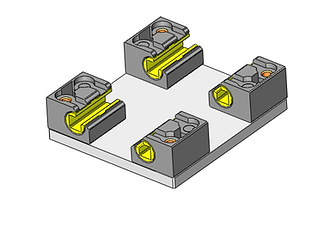top of page

## Transforming Table Project

Click these buttons to quickly scroll to the section.

Small apartments need furniture to be multifunctional and flexible. Who has space for a coffee table and dinner table and work table? What if the same table can transform between the three with a click of a button?

What Else Exists

The main difference between a coffee table and a dinner table is the height. Coffee tables are typically couch cushion height at 18-20 inches and dining/work tables are typically 30-32 inches.

One of the coolest coffee table to dining table transforming tables is the Boulon Blanc shown in the image on the right. A fully manual transformation, the table has two binary heights, and it uses a cable connection to coordinate the leg rotations. Boulon Blanc’s kickstarter page shows the transformation.

Sit to stand work desks are a more common type of transforming table. Several varieties and mechanisms like a vertical lift and cascade lift are reviewed in my first Seek and Geek.

Why should there be a new machine?

A new table design that automates the coffee table to dining table transition would make a functional centerpiece to any room. The Boulon Blanc is great, but needs a manual human to transform it and is difficult to transform with objects on top.

A beautiful and functional table should be able to transform with a vase of flowers on top (little vibration, planar, and lift some weight), statically hold the weight of dishes, books, and people leaning, and transform quickly and quietly.Problem

## Brainstorming & Thoughtshowering

BrainstormingStrategy and concept development should always be substantiated by the feasibility of underlying physics principles. After sketching some of these initial ideas in my design notebook I checked several of the most critical features: (aka the am I crazy check)

1. Geometry | How large does the table diameter need to be to allow three linear actuators enough range to raise and lower the table top the desired range from ~18 inches to 30 inches?

2. Forces & Stiffness | How large should the leg members be sized to support the forces of the table?

3. Motor Sizing | How much torque and power is required to lift the required forces?

Most of my calculations for this project are captured in excel models within one excel workbook. The most up to date calculation spreadsheet is posted here. Different calculations are included on separate tabs, and where relevant images of my hand calculations are included for reference. Calculations include:

• FRDPARRC Table

• Error Apportionment

• Stiffness and Structural Loop

• Force analysis and free body diagrams

Concepts

## Machine Design Specifications

Functional Requirements

This is a first pass FRDPARRC table start of the table for this transforming desk project. Further detail will be added as the concept is selected and engineering design continues.Forces & Stiffness

Identified in the FRDPARRC table above, two key functional requirements are that the table must be able to hold 120 lbs. statically and dynamically lift 20 lbs. in addition to the weight of the table itself, approximately 30 lbs.

Dynamically, the total is 50 lbs, which can be rounded to 25 kg of weight. The calculated dynamic force is 245 N, which can be rounded to 250 N dynamic force.

Statically, the total weight is 150 lbs., 68 kg, which yields a static force of 670 N which can be rounded to 700 N of static force.

Beyond simply being able to support the required static and dynamic loads, the table must maintain structural integrity and stiffness during actuation and static rest. The stiffness of the structure can be calculated by

F = k  x    ->    k = F / x

where F is the force of the desk, k is the desired stiffness of the structure, and x is the maximum allowable wiggle or desired accuracy.  As calculated the max force is 700 N and the the desired accuracy is 4 mm. The errors will be enumerated further in the error apportionment calculation. Thus, the overall structural stiffness is

k = 500 N / 0.004 m = 175,000 N/m = 1.75 x 10^5 N/m

Structural Loop

Tracing the structural loop of this transforming table, an estimate for the length of the structural loop is to multiply the sum of the distance each axis must travel by a factor of three.

This approximated structural loop can be approximated as a cantilevered beam with the length estimated for the structural loop.  Two scenarios can be calculated a circular tube cross section and a c-channel cross section. Assuming a circular tube cross section, where the outer diameter is 1/5 the length of the beam, and wall thickness is 1/20 the diameter of the beam, and for a c-channel that the width and height are both 1/5 beam length and wall thickness is 1/20 width, the stiffness of the beams can be calculated from F = k x, assuming that x is the deflection of the beam under a load. My calculations are shown below, and are included in my calculation spreadsheet under the Force and Stiffness tab. Comparing the three stiffnesses, it shows that the force accuracy method yields the lowest 10^5 and the c-channel is highest at 10^7.Natural Frequency

If the mass of the desk is N x the mass of the tube, what is a first order estimate of the natural frequency of the desk as a function of N? The natural frequencies can be determined referencing Roark's Formula for Stress and Strain (7th edition) (Equation 3b from Table 16.1, Chapter 16, page 765) Reference this link. Doing some algebra I found that

fn = (1/2pi) * sqrt (k / N*m_tube)Conservation of Energy Method

Understanding the necessary force of actuation of this design with estimates for the table loads is essential to validating the strategy and proceeding with more detailed concepts. If the actuation force necessary is too high then the high torque motor will become really expensive.## Linear Actuator Designbottom of page